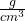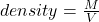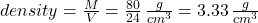## Density An object has a mass of 80 g. It is 3 cm wide, 4 cm tall and 2 cm long. What is the density of the object?​

Question

Density An object has a mass of 80 g. It is 3 cm wide, 4 cm tall and 2 cm long. What is the density of the object?​

in progress 0
2 months 2021-07-26T11:32:14+00:00 1 Answers 1 views 0

the density of the object is 3.33Explanation:

Use the formula for density:where M is the mass of the object, and V its volume.

In our example, we have:

Volume V = 3 x 4 x 2 cubic cm = 24 cubic cm

Then the density is: